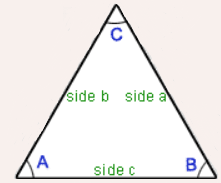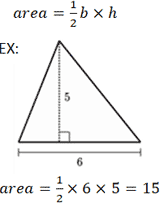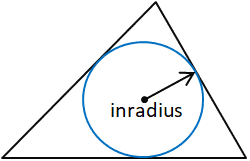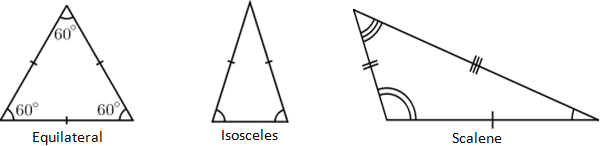# A Detailed Triangular(Triangle) Calculation#Triangle Calculator

This online angle calculator used to solves any triangles by entering the followings

2 sides and 1 angle (or)

2 angles and 1 side (or)

2 sides and 1 angle to find remaining values as used in trigonometry.## Enter 3 Positive Values and Click the "Calculate" button (Including at least one side)### Area of TriangleCalculating the area of a triangle involves its base, b, and height, h.

The base refers to any side of the triangle where the height is represented by the length of the line segment.

### Area of Inner RadiusInradius = Area / s

s = ( a + b + c ) / 2

where a, b, and c are the sides of the triangle

### Four Triangle Facts

1) It is not possible for a triangle to have more than one vertex with internal angle greater than or equal to 90°, or it would no longer be a triangle.

2) The interior angles of a triangle always add up to 180° while the exterior angles of a triangle are equal to the sum of the 2 interior angles that are not adjacent to it.

3) Another way to calculate the exterior angle of a triangle is to subtract the angle of the vertex of interest from 180°.

4) The sum of the lengths of any 2 sides of a triangle is always larger than the length of the 3rd side

### Types of Triangle

Equilateral triangle

The Equilateral triangle shown on the left has 3 congruent sides and 3 congruent angles.Each angle is 60°

Isosceles triangle

The Isosceles triangle shown on the left has 2 equal sides and 2 equal anglesScalene Triangle

The Scalene Triangle has no congruent sides. In other words, each side must have a different length.

Acute Triangle

The Acute Triangle has 3 acute angles (an acute angle measures less than 90°)

Obtuse Triangle

The Obtuse Triangle has an obtuse angle (an obtuse angle has more than 90°)

Since the total degrees in any triangle is 180°, an obtuse triangle can only have one angle that measures more than 90°.